# Math 2011 Student Edition Grade 3: Two dimensional shapes and their attributes Flashcards

Set Details Share
created 10 years ago by Piazza
1,155 views
Subjects:
math, education, elementary
Page to share:
Embed this setcancel
COPY
code changes based on your size selection
Size:
X

1

point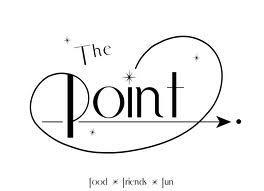an exact position often marked as a dot

2

line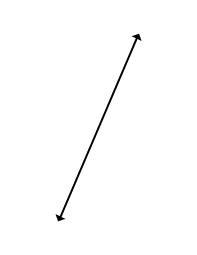a straight path of points that is endless in two directions

3

line segment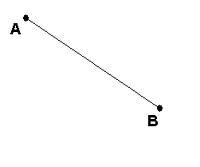a part of a line with two endpoints

4

intersecting lines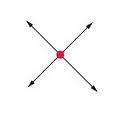lines that cross at one point

5

parallel lines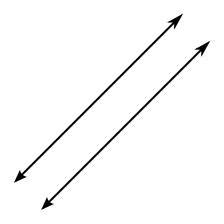lines that never cross

6

ray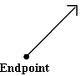a part of a line that has one endpoint and continues endlessly in one direction

7

angle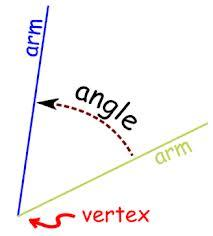a figure formed by two rays that have the same endpoint.

8

vertex plural: vertices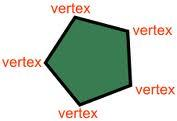1. the point where two rays met to form an angle.
2. the points where the sides of a polygon meet
3. the points where 3 or more edges meet in a
solid figure that does not roll.
4. the pointed part of a cone

9

right angle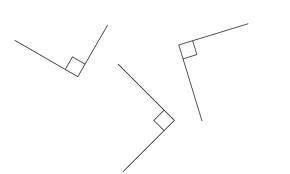an angle that forms one square corner or 90 degree angle

10

acute angle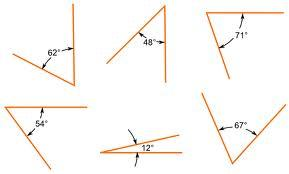an angle that's open end measures less than a right angle

11

obtuse angle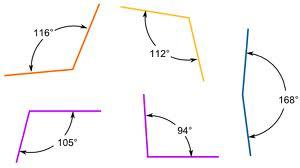an angle that's open measures more than a right angle

12

perpendicular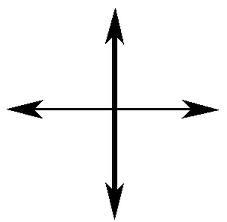two lines, line segments or rays that form right angles

13

polygon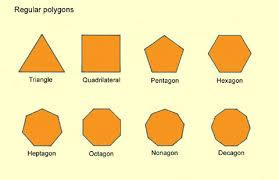a closed figure made up of line segments

14

side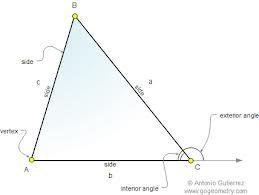a line segment forming part of a polygon

15

triangle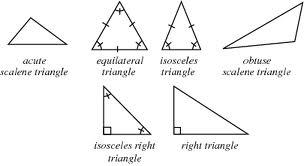a polygon with 3 sides and 3 vertices

16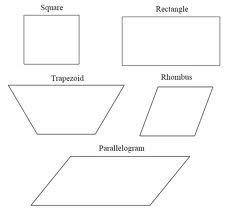a polygon with 4 sides and 4 vertices

17

pentagon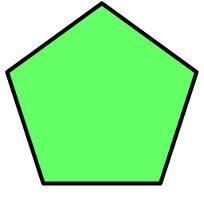a polygon with 5 sides and 5 vertices

18

hexagon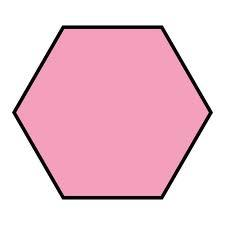a polygon with 6 sides and 6 vertices

19

octagon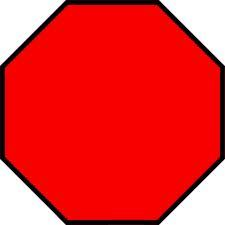a polygon with 8 sides and 8 vertices

20

decagon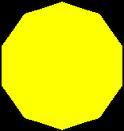a polygon with 10 sides and 10 vertices

21

equilateral triangle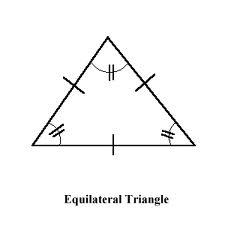a triangle with all sides the same length

22

isosceles triangle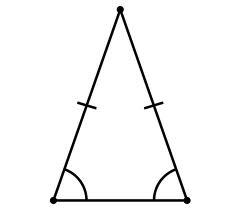a triangle with at least two sides the same length

23

scalene triangle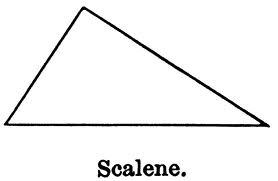a triangle with no sides the same length

24

right triangle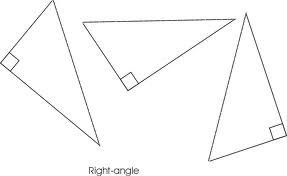a triangle with one right angle

25

acute triangle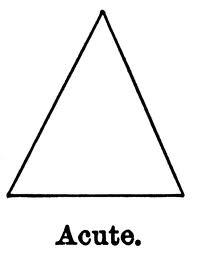a triangle with 3 acute angles

26

obtuse triangle

a triangle with one obtuse angle

27

trapezoid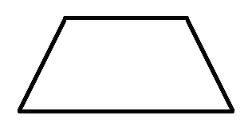a quadrilateral with only one pair of parallel sides

28

parallelogram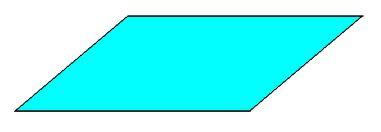a quadrilateral in which opposite sides are parallel

29

rectangle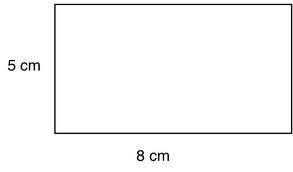a quadrilateral with four right angles. It is a special parallelogram

30

rhombus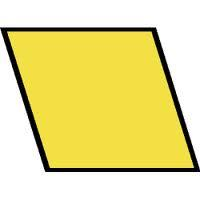a quadrilateral with opposite sides parallel and all sides the same length. Opposite angles are obtuse and the other opposite angles are acute. It is a special parallelogram

31

square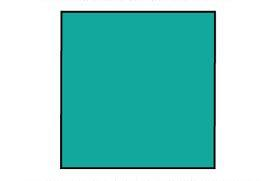a quadrilateral with 4 right angles and all sides the same length. it is a special parallelogram and a combination of a rectangle and rhombus

32

diagonal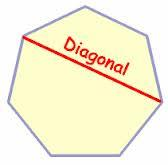a line segment other that a side that connects two vertices of a polygon